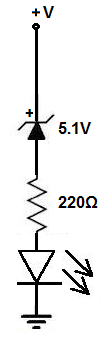﻿ How to Build Voltage Level Indicator Circuit with a Zener Diode ﻿# How to Build a Voltage Level Indicator with a Zener DiodeIn this circuit, we will show how to build a voltage level indicator with a single zener diode.

A voltage level indicator is a circuit which can show if the voltage input into a circuit is greater than a certain threshold level. If the voltage is greater than a certain level, then an output such as an LED can light up or a buzzer can sound off.

We use a single zener diode in this circuit to function as our voltage level indicator.

A zener diode is a diode which has a break downs when a certain voltage level is reached and conducts current- when it is connected reverse biased in a circuit. This voltage that causes current to conduct across it is called the breakdown voltage of the zener diode. If a zener diode has a breakdown voltage of 5.1V, after 5.1V or greater is applied to it, it will conduct current across it which can then power on a load.

In this circuit, the type of zener diode we will use is the 1N4733 zener diode, which has a breakdown voltage of 5.1V. The type of output we will use is an LED.

So when the voltage reaches above the threshold of 5.1V, the diode conducts current across and lights up the LED, indicating the voltage level.

Components Needed

• 1N4733 5.1V Zener Diode
• 220Ω resistor
• LED

The 1N4733 zener diode can be obtained very cheaply from a number of online retailers. It can be obtained from Tayda Electronics at the following link: 1N4733 5.1V Zener Diode.

The datasheet for this diode can be found at: 1N47XX Zener Diode Datasheet.

This diode again has a breakdown voltage of 5.1V. After 5.1V, it will break down and conduct current across from cathode to anode.

The pinout for a zener diode is shown below:The cathode end of the zener diode is the end with the band on it. The other end is the cathode.

### Voltage Level Indicator Circuit

The voltage level indicator circuit we will build with a 1N4733 zener diode is shown below.The breadboard schematic of the above circuit is shown below.So the circuit is very simple.

The zener diode is connected in reverse biased to the power source. After this comes the 220Ω resistor that limits current to the LED and then we have the LED.

How this circuit works is the power source feeds voltage to the circuit.

When the voltage goes above the 5.1V threshold, then the zener diode breaks down and begins to conduct current across from its cathode to its anode. This current then powers on the LED, lighting it up. The LED serves as a visual indicator that the voltage that is being fed into the circuit is greater than 5.1V, the breakdown voltage of the 1N4733 zener diode.

Be aware that since the LED needs requires a forward voltage across it, normally of about 2-3V in order to turn on, the LED won't turn on until the voltage fed into the circuit is about 7-8V. This is because the zener diode consumes 5.1V and then the LED needs about 2-3V of forward voltage in order to turn on. So this circuit really is an indicator that the voltage in the circuit is greater than 7V. If the LED is on, then the voltage is definitely greater than 5.1V, which is the breakdown of the zener diode. But since you have to take into account the voltage needed for the LED to turn on, you have to add that voltage amount. Then you can truly see the level the volage is at. Of course, if you're just building a circuit to see if the voltage exceeds the breakdown voltage of a zener diode generally (by a few volts or so), then you don't need to worry too much about the forward voltage that an LED consumes.

And you can make many modifications to this circuit. You can swap out this zener diode for another to get a different voltage threshold.

The types of zener diodes you can use are:

1N4728: 3.3V

1N4729: 3.6V

1N4730: 3.9V

1N4731: 4.3V

1N4732: 4.7V

1N4734: 5.6V

1N4735: 6.2V

1N4736: 6.8V

1N4737: 7.5V

1N4738: 8.2V

1N4739: 9.1V

1N4740: 10V

1N4741: 11V

1N4742: 12V

1N4743: 13V

1N4746: 18V

These are many different zener diodes you can use. They all have different breakdown voltages, so they can set a different threshold voltage for the voltage level indicator circuit.

And this is how a voltage level indicator can be built with a zener diode.

To see how this circuit works in real life, see the following video shown below.

Related Resources

How to Build a Zener Diode Voltage Regulator

How to Build a Voltmeter Circuit with Zener Diodes